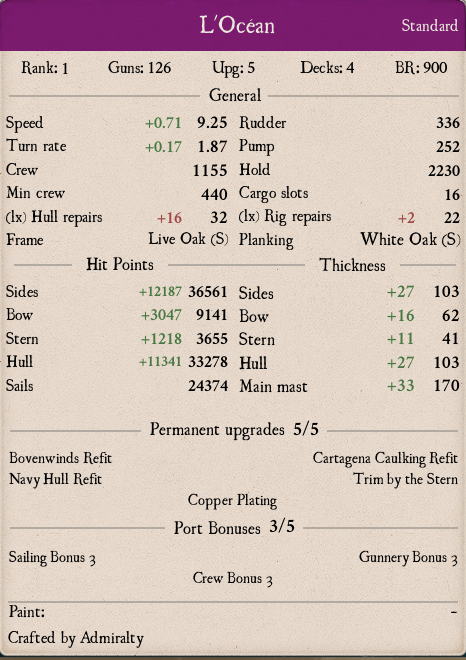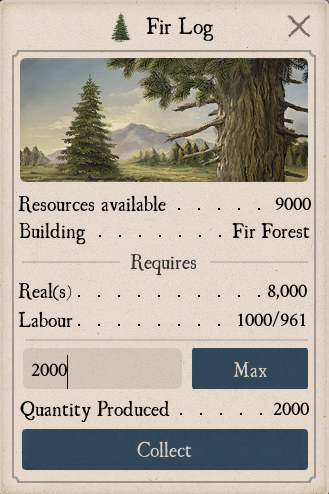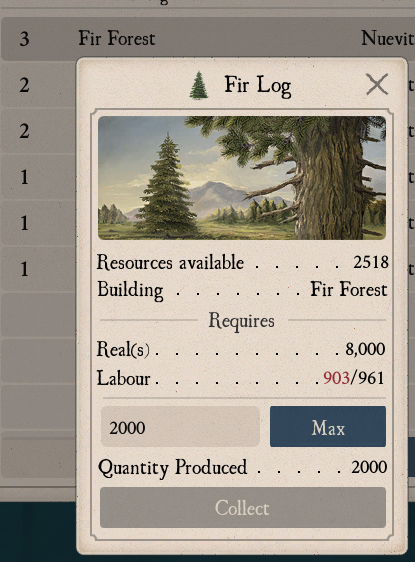Game-Labs Forum

## Recommended Posts

Posted (edited)

So I was doing some trivial calculations to figure out the impact of wood price change when I stumbled on this problem

2000 * A  = 961

I was  trying to figure out the price of a ship in BOTH reals and Labor Hours.

There is 2 ways you can reduce amount of Labor Hours:

1. Increasing your "Farm" level to 2 and 3 to get 10% and 20% LH reduction respectivaly.
2. Having 40% Labor Hours Reduction activated in your port

In lvl1 Fir Farm 1 fir log requires 1 Labor Hour

1 fir = 1 LH

So, Lets say you have Fir Farm lvl3 AND you have your LH Reduction activated.  Thats 20% and 40% reduction right? The most simple way to calculate it would be:

A * (1-(B+C)) = D ,where

• A is a number of Fir Logs that we want to extract AND the base amount of LH that you will need
• B is LH reduction provided by FIR LVL3 bonus
• C is LH reduction  provided by port LH Reduction bonus
• D is your result - the Amount of Hours you will need after applying all reductions to A

Trying to get 2000 fir logs using this approach will give you following result

2000 * ( 1-(40% + 20% )) = D

2000 * (1-(0.4 + 0.2)) = D

2000 * 0.4 = 800

Simple math. 2000 fir logs would require me 800 Labor Hours, because I have 60% LH reduction

if you arent really good in such basic math I could even proove it for you:

• 100% - 40% = 60%
• 60% we can write it down as 40% + 40%/2
• We know that 40% is 800
• 60% = 800 + 800/2 = 800+400=1200
• 60% + 40 % = 100%
• 1200 + 800 = 2000

Case solved. This topic now might be closed by moderators. Right?

WRONG!

And by the way.

The person who will manage to convice me that his answer IS RIGHT shall recive this beautifull shipEdited by Beeekonda
•5

##### Share on other sites
Posted (edited)

So I've spent some time trying to figure out the exact way LH discounts works.

First thing I did is tried to figure out how much Labor Hours it actually takes to produce 1 fir log by trying different inputs. LVL3 Farm + Port Bonus

• 1 log = 1 hour
• 2 logs =  1hours
• 3 logs = 2 hours
• 4logs = 2 hours

X logs = X/2 Hours will continue till 40 when this will no longer be a case.

• 40 logs = 20 hours
• 50 logs = 24 hours
• 60 logs = 29 hours
• 70 logs = 34 hours
• 80 logs = 39 hours
• 90 logs = 44 hours

I was doing this untill I got to 2000 logs

2000 logs = 961 hours

2000 logs (which is also 2000 BASE LH needed) gets a reduction from the bonuses becomes 961

HOW?

After spending over an hour trying to figure this out I found my LVL2 Fir Farm on my alt and tried to get 2000 logs out of it:

2000 logs = 1080 hours   -  LVL2 gives 10% reduction and PORT BONUS gives 40% I started working on this thing

First lets write it down as somewhat equasion looking

X * Y = Z

2000 * Y = 1080

Y = 1080/2000

Y = 0.54

and 2000 multiplied by 0.54 is indeed  1080

Now we need to figure out how 10% and 40% (or 0.1 and 0.4) turned out to be 0.54

(1 - 0.1) * (1 - 0.4) = x

0.9 * 0.6 = 0.54

So, LVL2 farm + Port Bonus:

2000 * (1-0.1) * (1-0.4) = x

2000 * 0.9 * 0.6 = x

x = 0.54

Then I did the same for lvl3 farm and bonus

2000 * (1 - 0.2) * (1 - 0.4) = x

2000 * 0.8 * 0.6 = x

x = 960

Edited by Beeekonda
•2
•1

##### Share on other sites
Posted (edited)

So by multiplying amout of resources we want by the % of each labour reduction we get the answer, right?

WRONG!

First we make a shortcut

10% and 40% will be  (1 - 0.1) * (1 - 0.4) = 0.54

20% and 40% will be (1 - 0.2) * (1 - 0.4) = 0.48

so, for lvl3 and bonus

60 logs is 60 * 0.48 = 28.8 hours and it rounds up to 29

90 logs is 90 * 0.48 = 43.2 hours and it rounds up to 44. We are being ripped off here but ok. At least it makes SOME sense.

2000 logs is 2000 * 0.48 = 960 HOURS!!!!! ITS NINE HUNDRED SIXTY.  NO DECIMAL.

SO HOW THE HELL IT BECOMES 961?pls expain

Edited by Beeekonda
•2

##### Share on other sites
Posted (edited)

I’m not sure what you’re looking for. I am a long way from learning or using much math, but...

The adjustments to labor are applied separately.

First, the port’s reduction:

2000 x .6 = 1200

1200 x .8 = 960

I assume somewhere in there is a rounding up to 961.

I really hope I don’t now have to copy and paste this on my Facebook page and tag my friends.

Edited by Farrago
•1

##### Share on other sites

Aw, relax. Everything will be okay once we have wind shadow in game.

##### Share on other sites•1

##### Share on other sites
Posted (edited)

Because game use floating-point types

Try this code (I use csc.exe (Microsoft (R) Visual C# Compiler version 4.7.3062.0))

```using System;

namespace App
{
class Program
{
static void Main(string[] args)
{
double a=1 - 40*0.01f;
double b=1 - 20*0.01f;
Console.WriteLine("2000*0.6*0.8={0} ({1})",2000*a*b, Math.Ceiling(2000*a*b));

}
}
}```

Result

2000*0.6*0.8=960,000019669533 (961)

Math.Ceiling - Returns the smallest integral value greater than or equal to the specified number.

But if double (Precision ~15-17 digits) replace to float (Precision ~6-9 digits) then we get 960

Edited by qw569😳
•3

##### Share on other sites
Posted (edited)

For some reason NA decided it was 48% discount.

Why?

Because it's naval action. Need anything else be said.

P.s. they take the 20% discount once the 40% has been applied. Because naval action. Then They break basic rules of mathematics and round up. Because naval action.

(2000-(2000x0.4)) - ((2000-(2000x0.4))x0.2

= 960

960 + NA = 961

Edited by RepairyMcRepairous
•1

##### Share on other sites

960 hours of virtual labor and 1 hour of virtual grind?

##### Share on other sites
9 hours ago, qw569😳 said:

Because game use floating-point types

Let me know when you want to get your ship##### Share on other sites
1 hour ago, Beeekonda said:

Let me know when you want to get your shipthanks, leave the ship to yourself

•1

## Join the conversation

You can post now and register later. If you have an account, sign in now to post with your account.
Note: Your post will require moderator approval before it will be visible.×   Pasted as rich text.   Paste as plain text instead

Only 75 emoji are allowed.